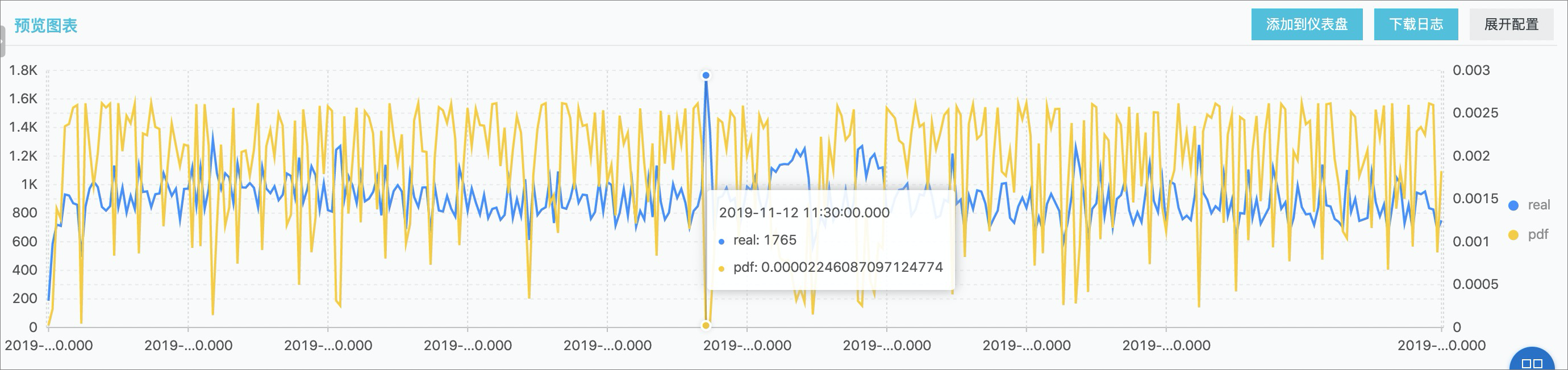• 函数格式
``select kernel_density_estimation(bigint stamp, double value, varchar kernelType)``
• 参数说明
参数 说明
stamp UnixTime 时间戳数据，单位为秒。
value 对应的观测数值。
kernelType
• box：矩形。
• epanechnikov：Epanechnikov 曲线。
• gaussian：高斯曲线。
• 输出结果
显示项 说明
unixtime 对应原始数据的时间数据。
real 对应的观测数值。
pdf 对应的每个观测点的概率值。
• 示例
• 代码示例：
``````* |
select
date_trunc('second', cast(t1 as bigint)) as time, t1 as real, t1 as pdf from (
select kernel_density_estimation(time, num, 'gaussian') as res from (
select __time__ - __time__ % 10 as time, COUNT(*) * 1.0 as num from log group by time order by time)
), unnest(res) as t(t1)  limit 1000``````
• 结果示例：